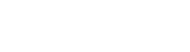# Compound Interest CalculatorCASAPLORERTrusted & Transparent

Our compound interest calculator determines your total savings and interest earned over a period of time. Compound interest is the process of earning interest on both the principal and the accumulated interest from preceding periods. The total savings will consist of the capital and all the interest earned.

\$
\$
Frequency
Years
%
Total Investment
\$35,365
Total Interest
\$24,365
Total Principal
\$11,000

## How do I Calculate Compound Interest?

In order to understand compound interest, it is essential to know another concept known as simple interest. Simple interest is the process of your initial principal earning interest, however, only the initial principal earns interest and not the interest you earned previously.

### Simple Interest Formula• A = Final Amount
• P = Initial Principal
• r = interest rate
• t = Time Period
Requirements
• Initial Principal = \$10,000
• Time Period = 20 years
• Interest Rate = 5%
Results
• Total Investment = \$20,000
• Interest Earned = \$10,000
• Compound interest on the other hand earns you interest on the initial principal and any interest you earn from that point forward. This allows you to save money faster as over time, the interest you earn is on a larger capital base.

### Compound Interest Formula• A = Final Amount
• P = Initial Principal
• r = Interest Rate
• t = Time Periods Elapsed
• n = Number of Times Interest is Applied
Requirements
• Initial Principal = \$10,000
• Time Period = 20 years
• Interest Rate = 5%
• Compounding = Annually
Results
• Total Investment = \$26,533
• Interest Earned = \$16,533
• ## What is a Compounding Period?

The compounding period determines when the interest is compounded on your total capital. For example, the most common compounding period is annual compounding where a year needs to pass for the interest to compound again. Once the compounding period is over, interest is added to the capital. In the next compounding period, more interest will be earned on the larger capital. Our calculator has the ability to do daily, quarterly, semi-annual and annual compounding.

Any calculators or content on this page is provided for general information purposes only. Casaplorer does not guarantee the accuracy of information shown and is not responsible for any consequences of its use.